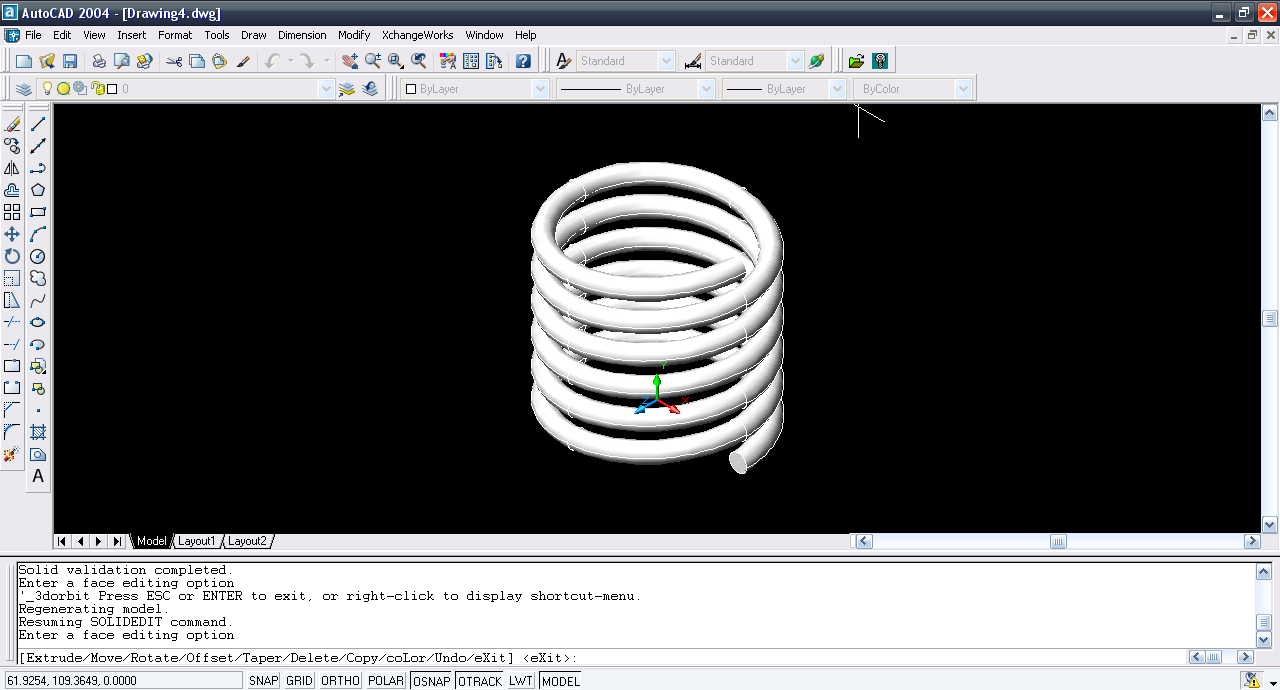# Tutorial - Modeling Spring in AutoCAD using extrude face?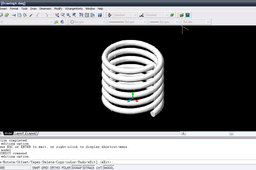Here is the tutorial.

1. ### Step 1: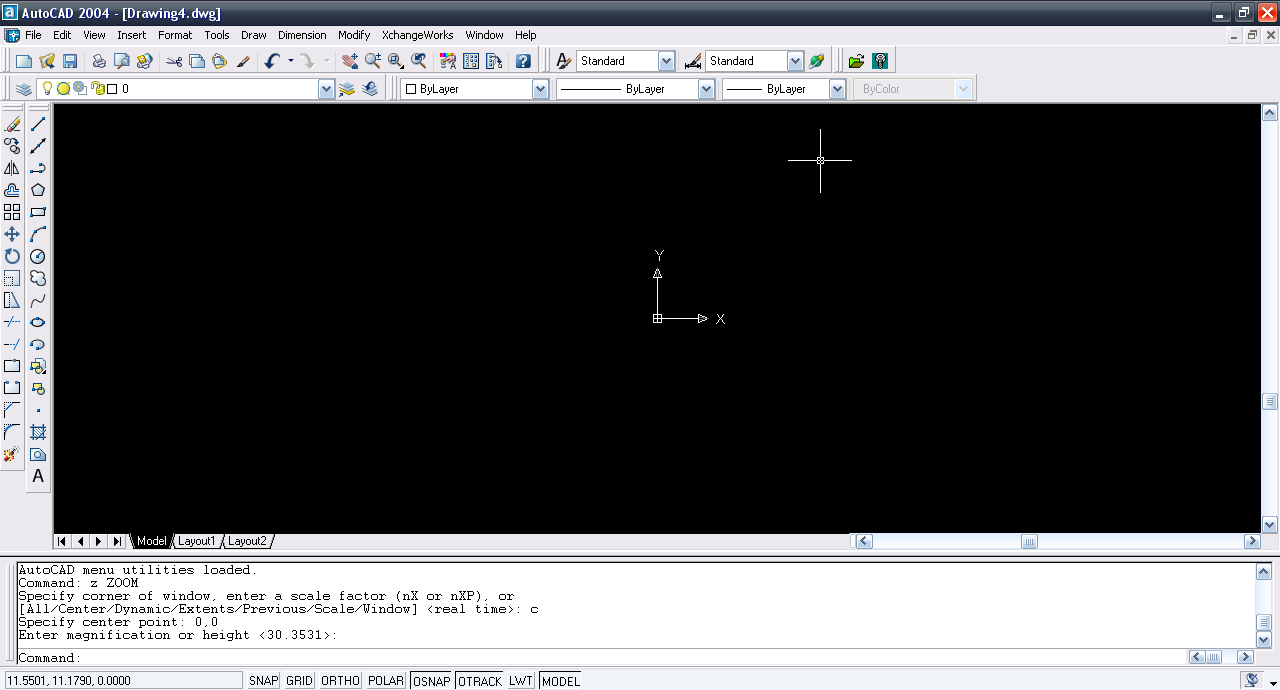2. ### Step 2:

Draw a circle.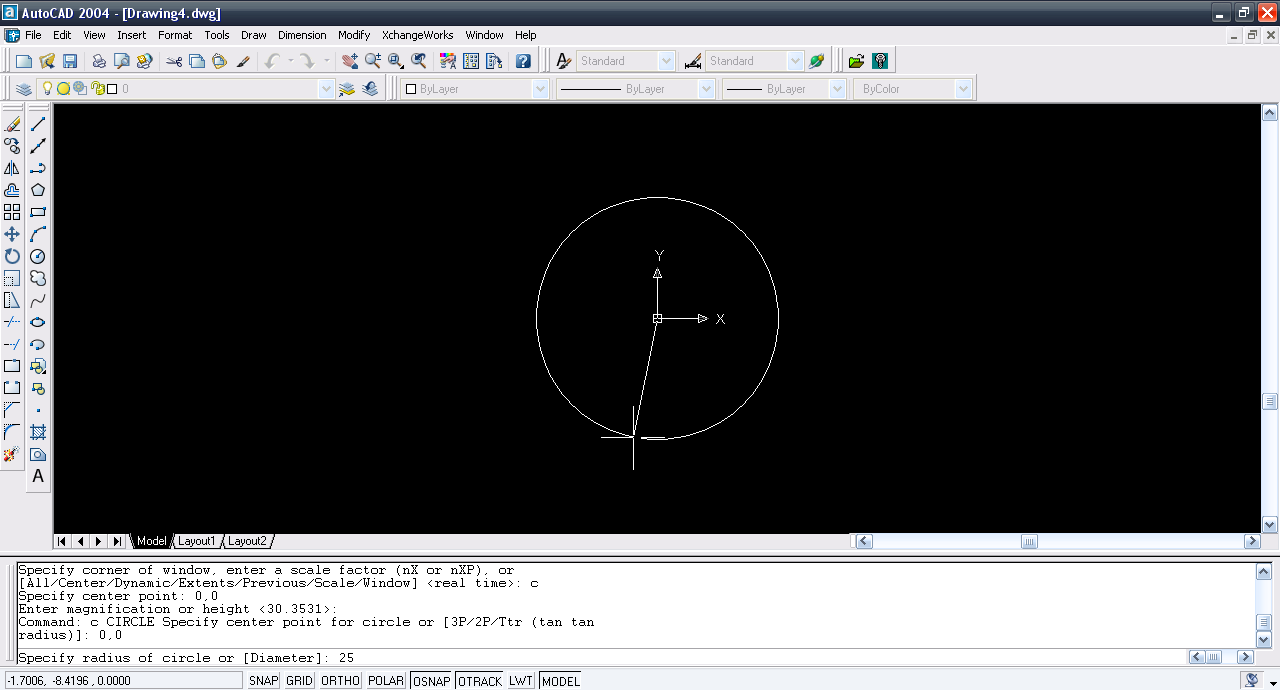3. ### Step 3:

Draw a line bisecting the circle in half.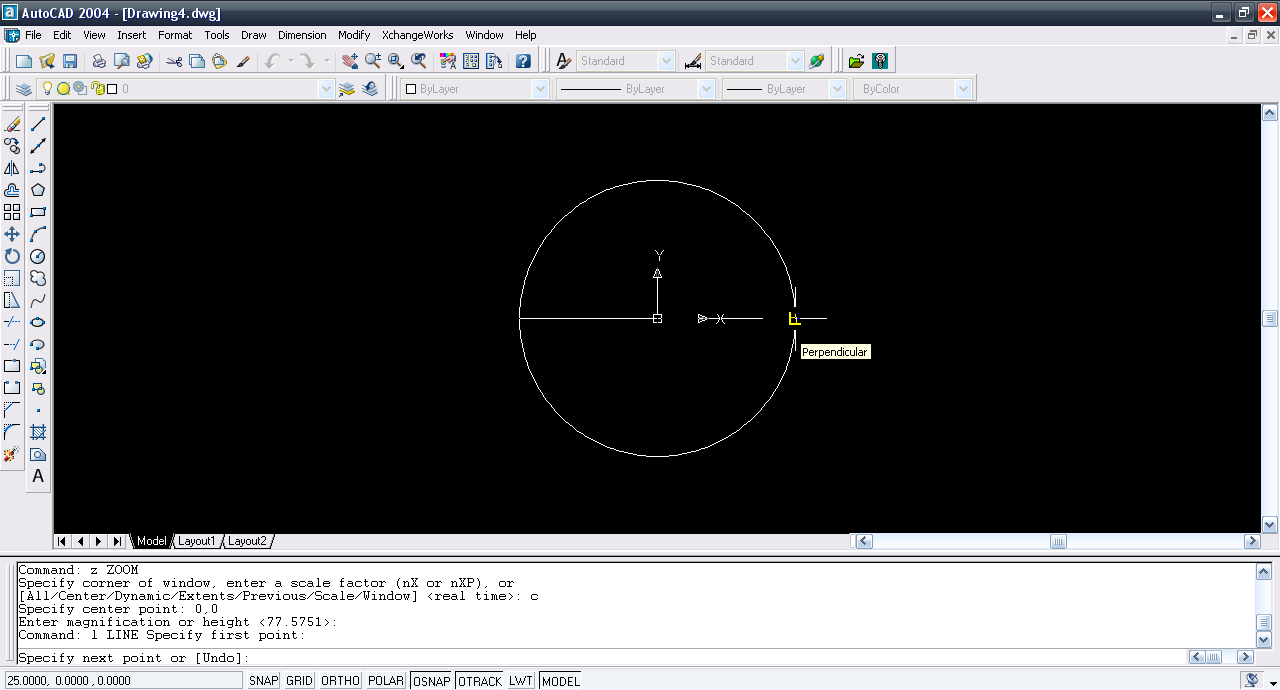4. ### Step 4:

Select trim tool. Select all.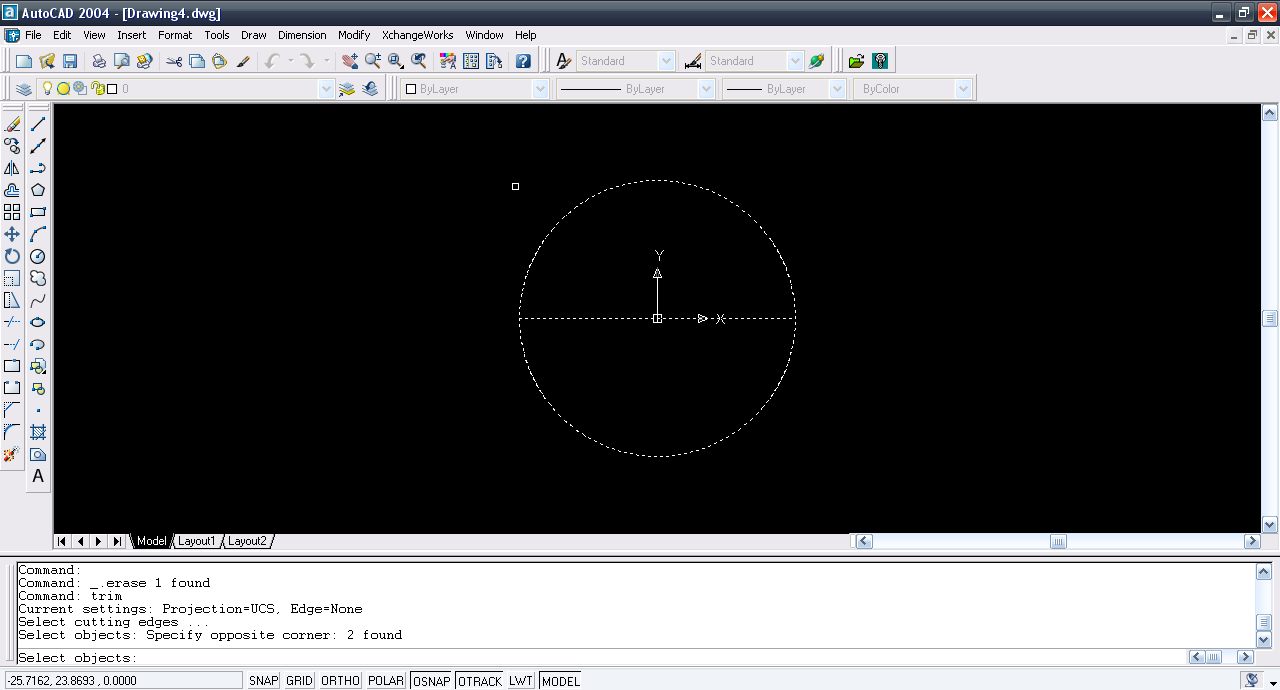5. ### Step 5:

Trim the lower half.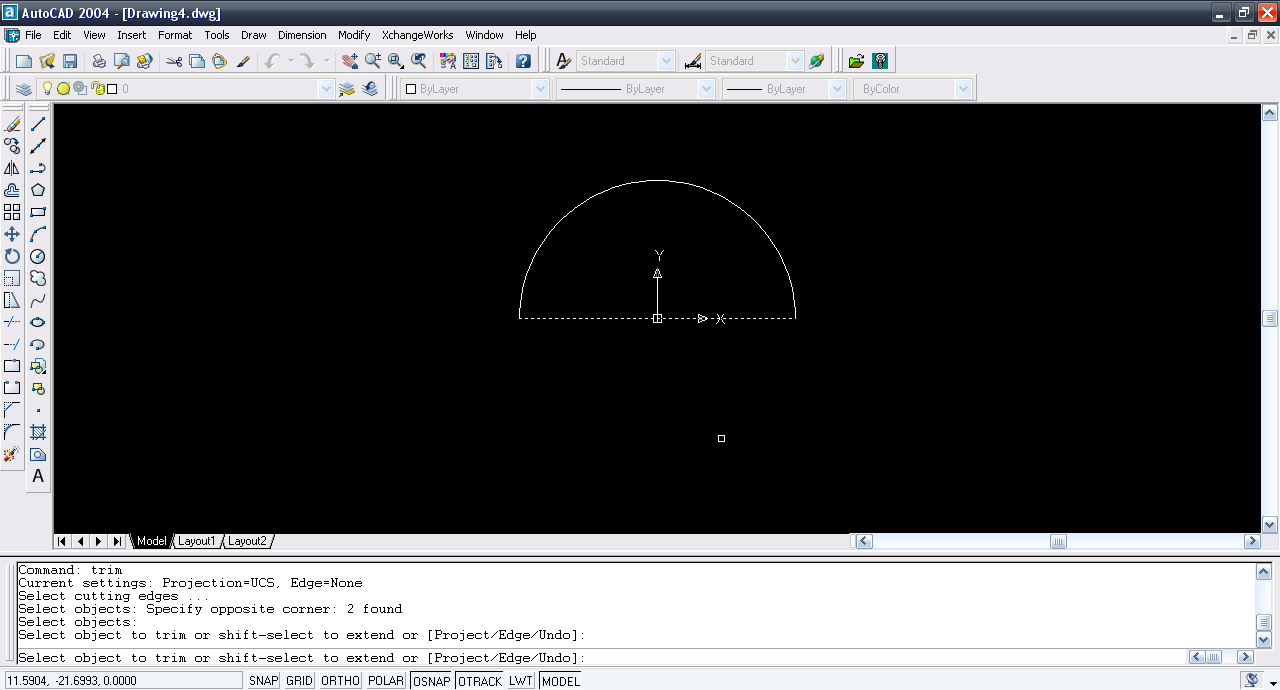6. ### Step 6:

Erase the line. Now use mirror command and select the circle.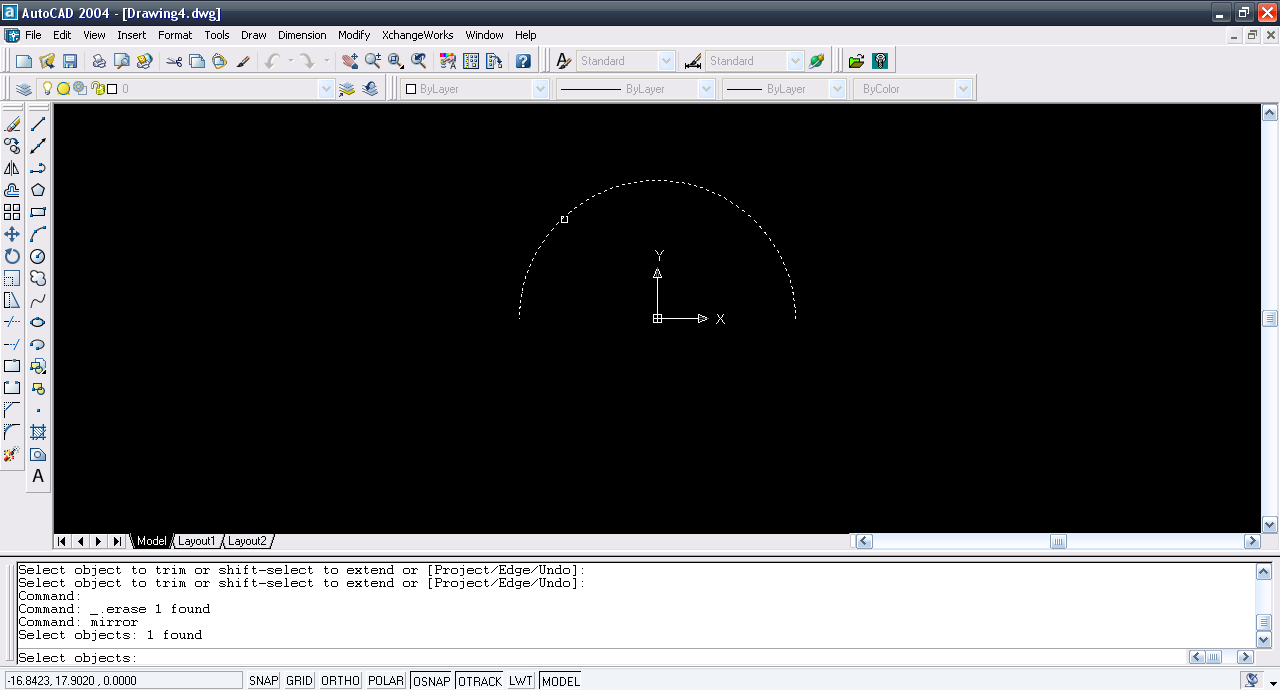7. ### Step 7:

Mirror it along the arc end points.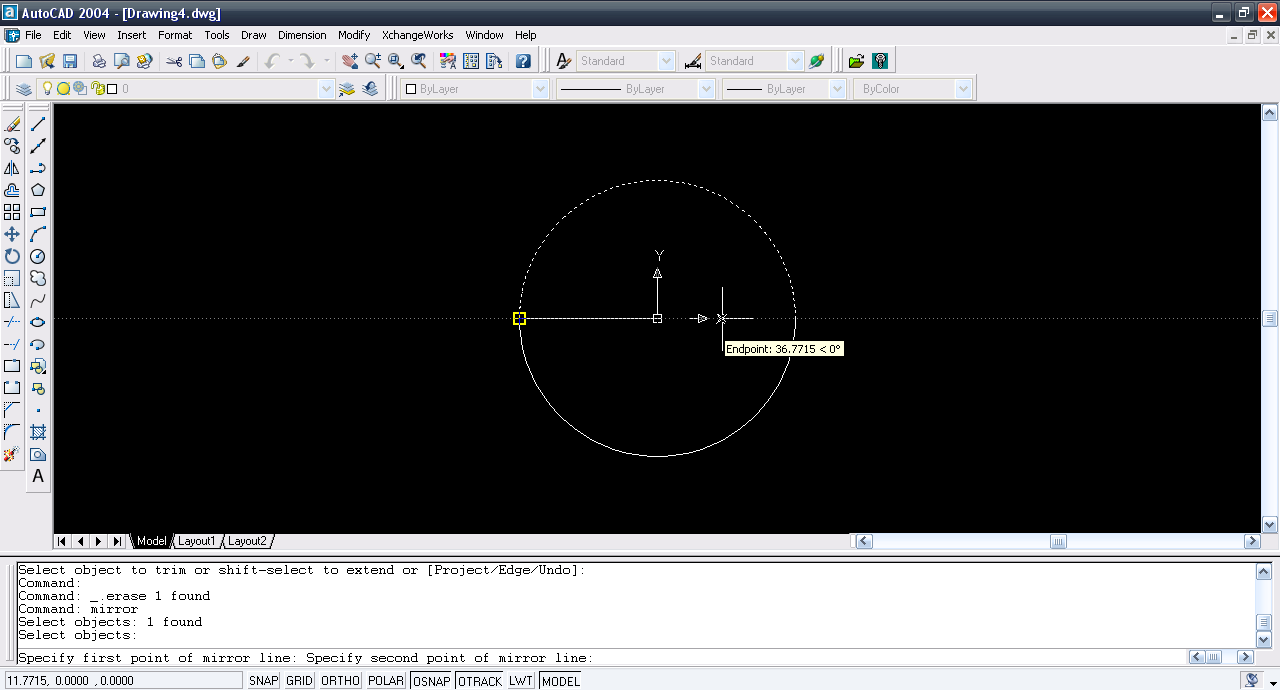8. ### Step 8:

Use viewpoint command and enter 1,-1,1 or choose SE Isometric under view menu.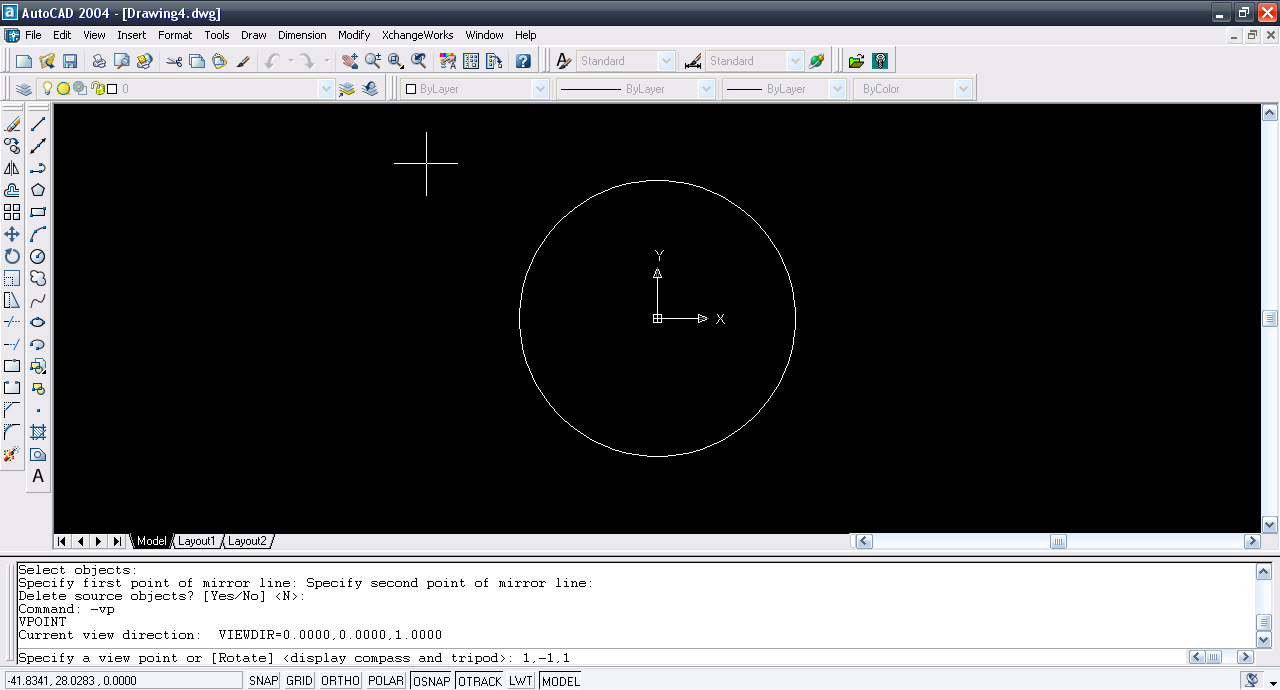9. ### Step 9:

Rotate3d and then select the half circle.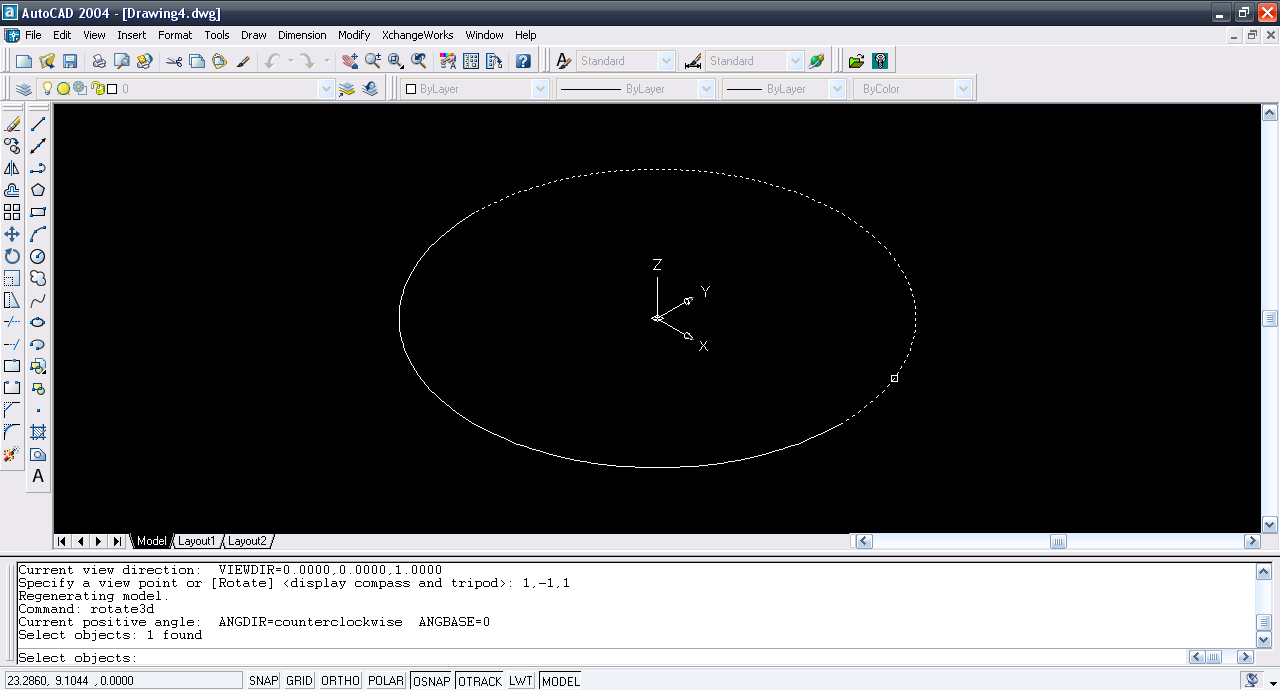10. ### Step 10:

Select Y-axis.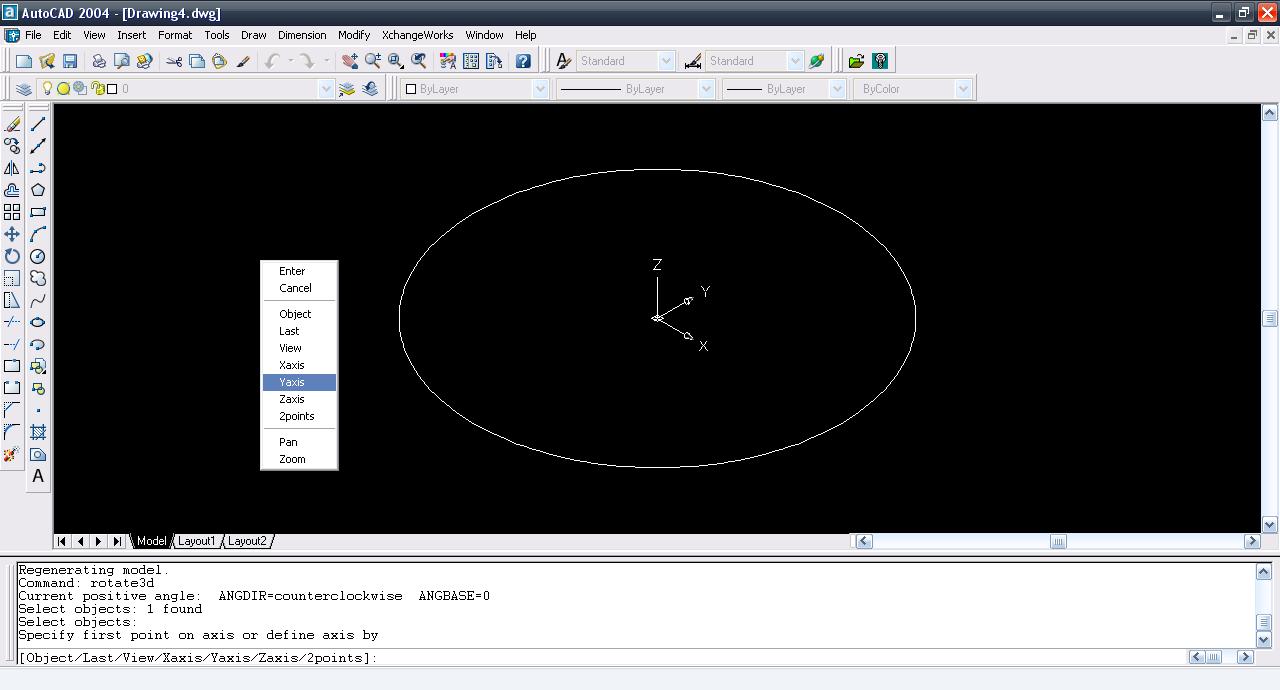11. ### Step 11:

Select the end point of the arc and rotate it by 5º.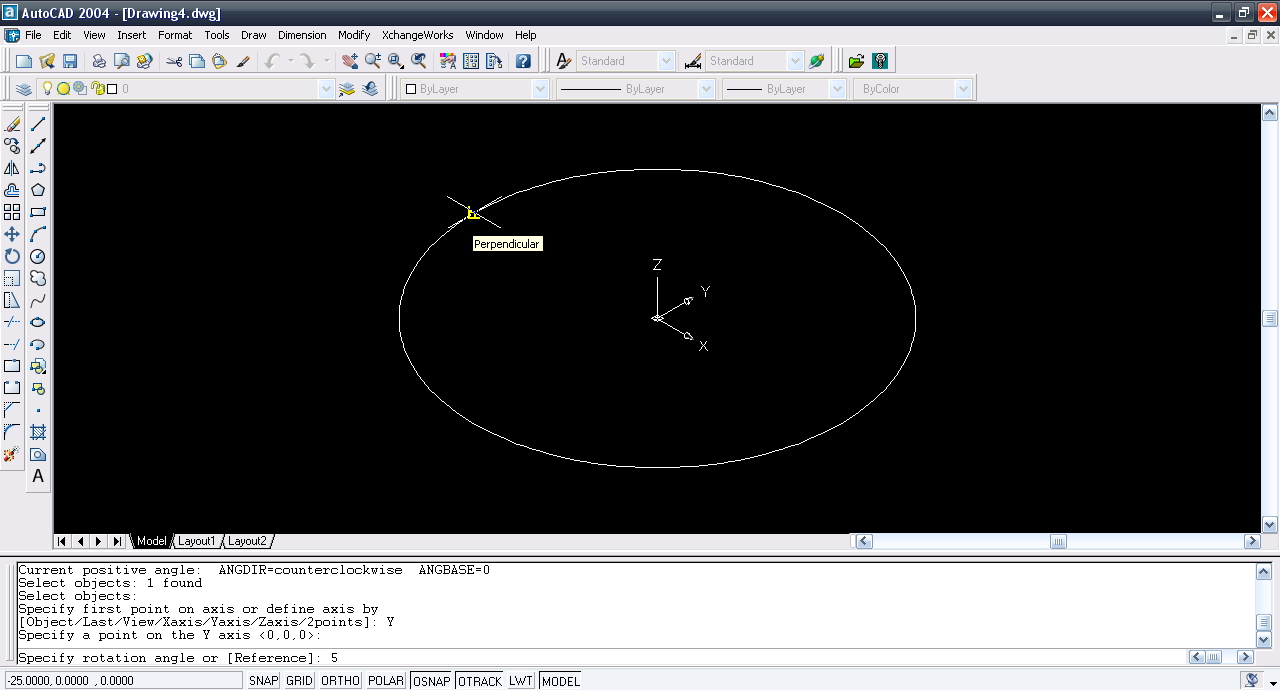12. ### Step 12:

Repeat the same step for the other half.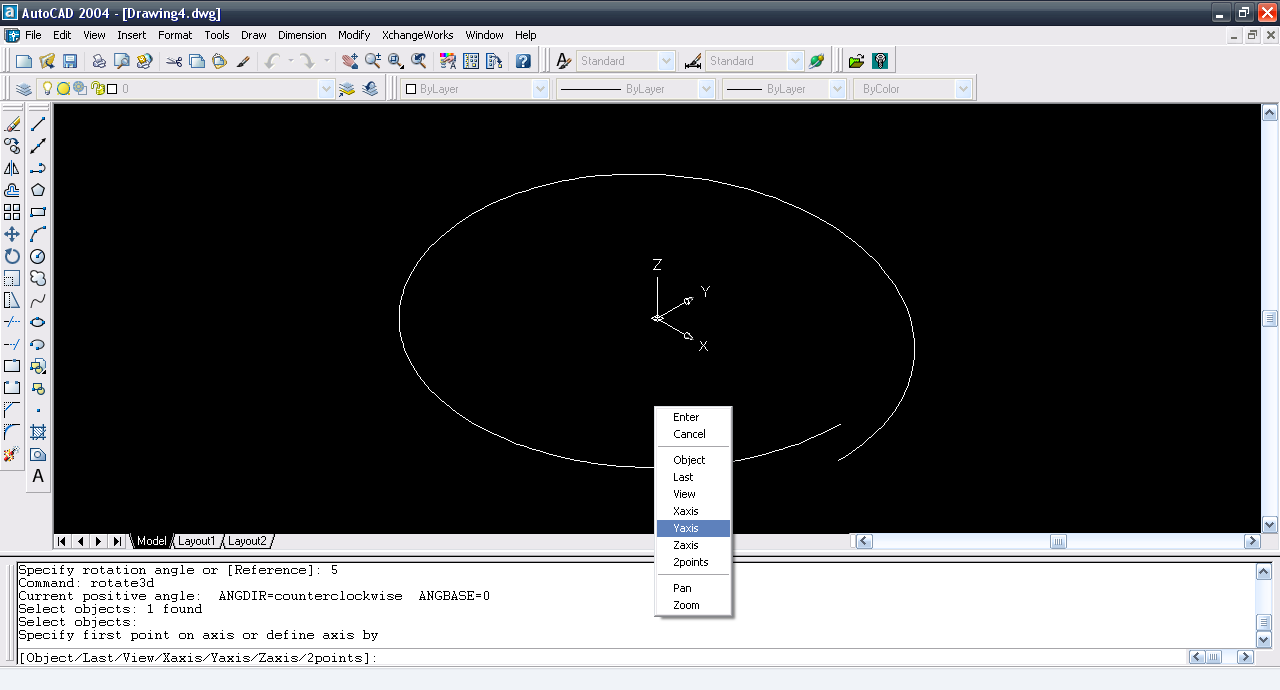13. ### Step 13:

Rotate it by -5º.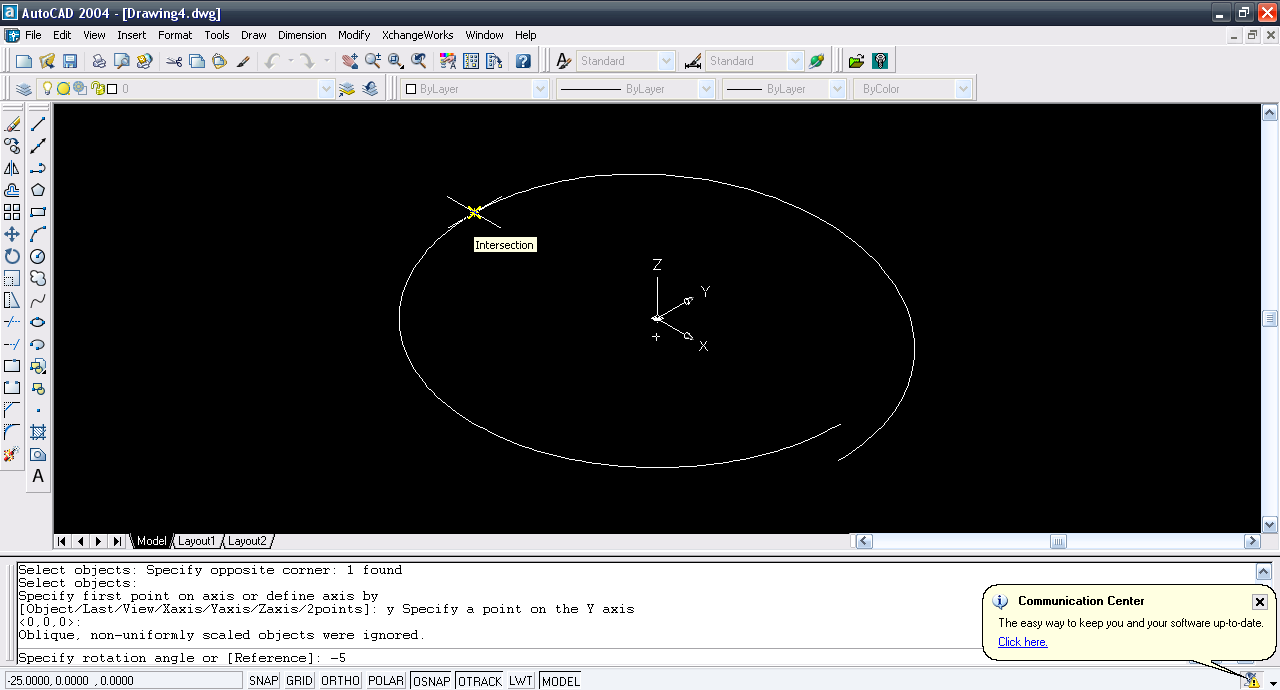14. ### Step 14:

Copy command and then select both arc.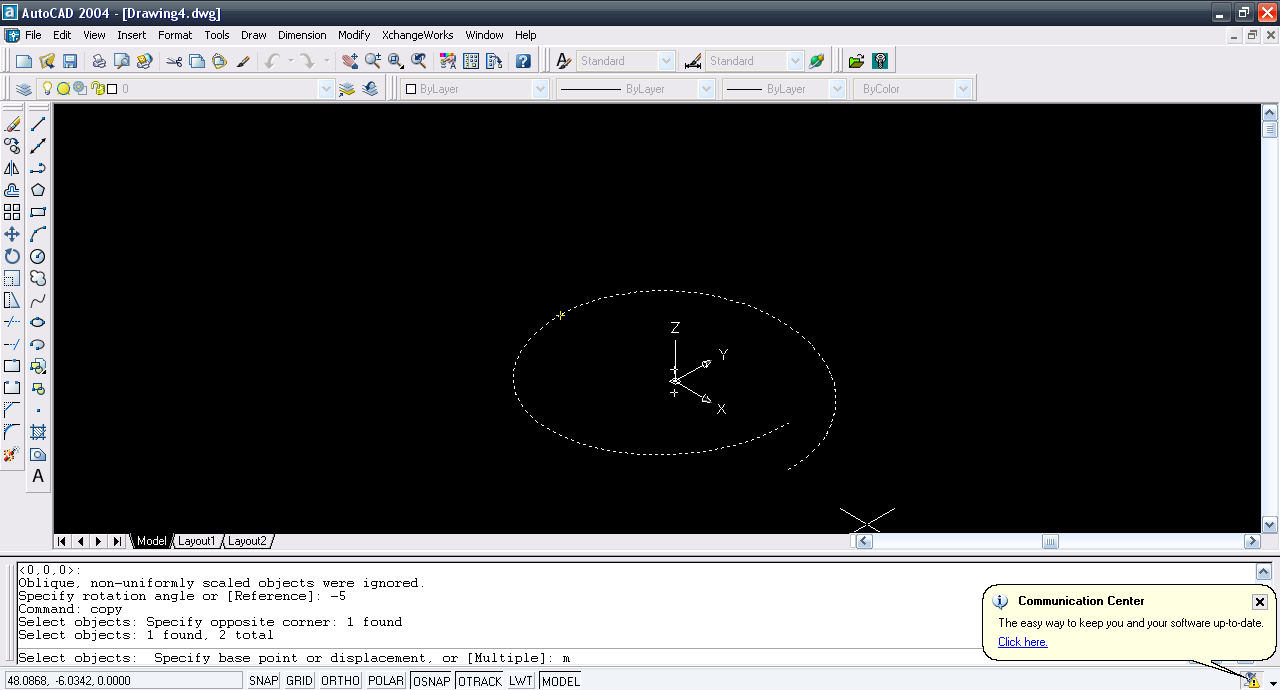15. ### Step 15:

Multiple type copy. Select the lower end point as the base point.16. ### Step 16:

Selct the upper point as target point.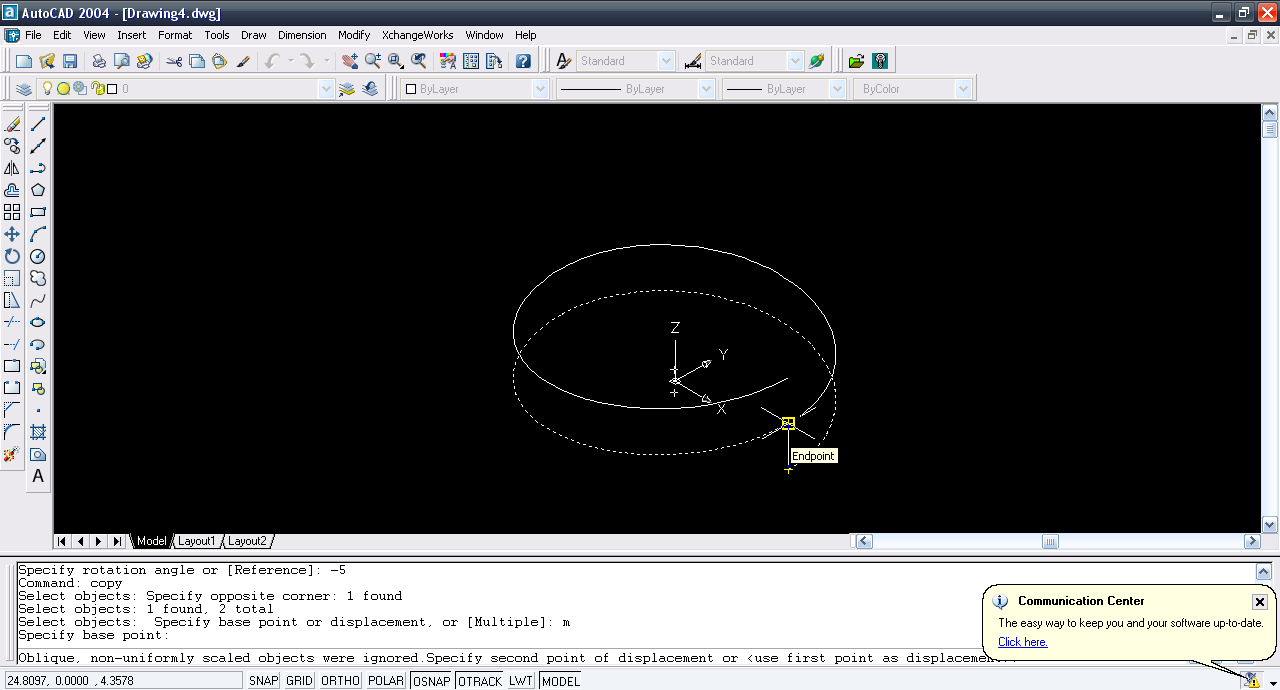17. ### Step 17:

Repeat the same step and we have helix.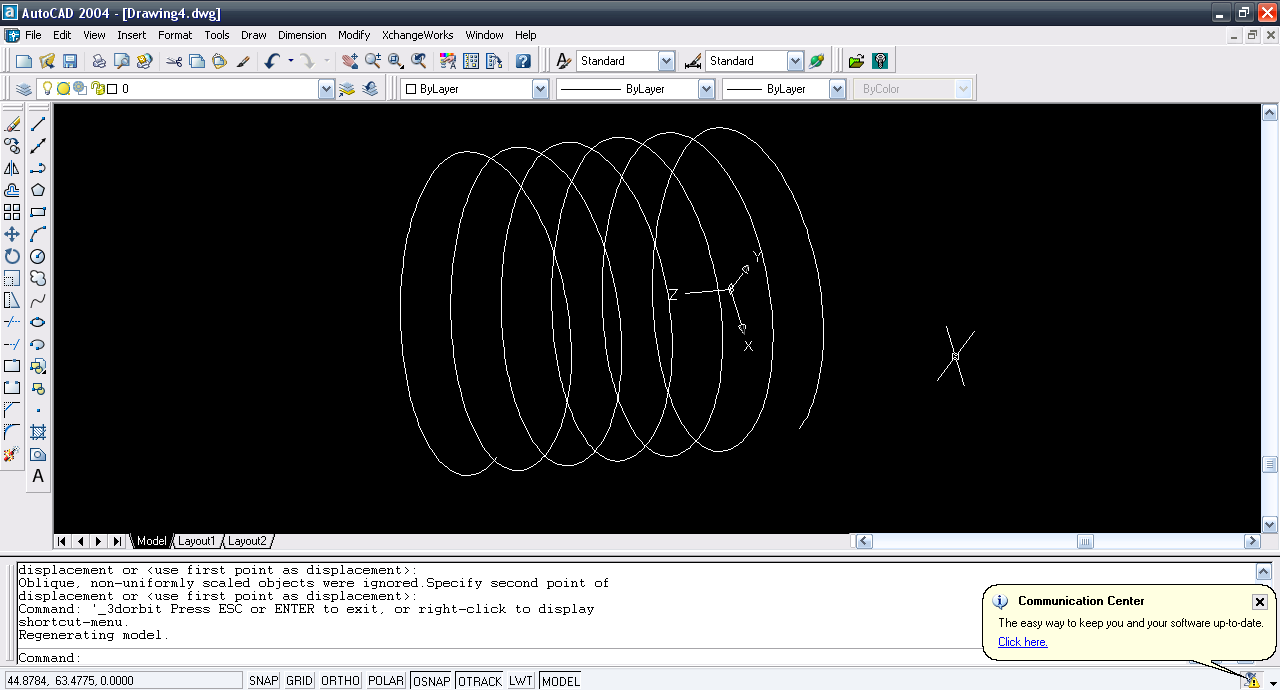18. ### Step 18:

Change the UCS about X at 90º.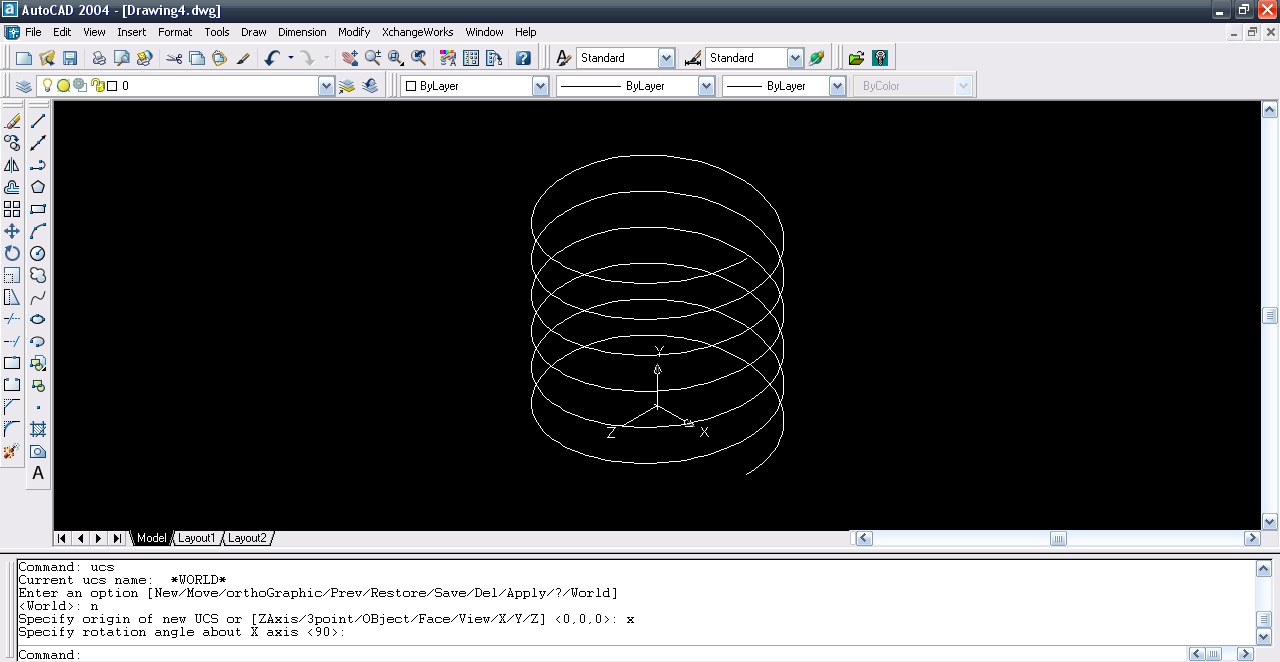19. ### Step 19:

Draw a circle at the end point of the helix.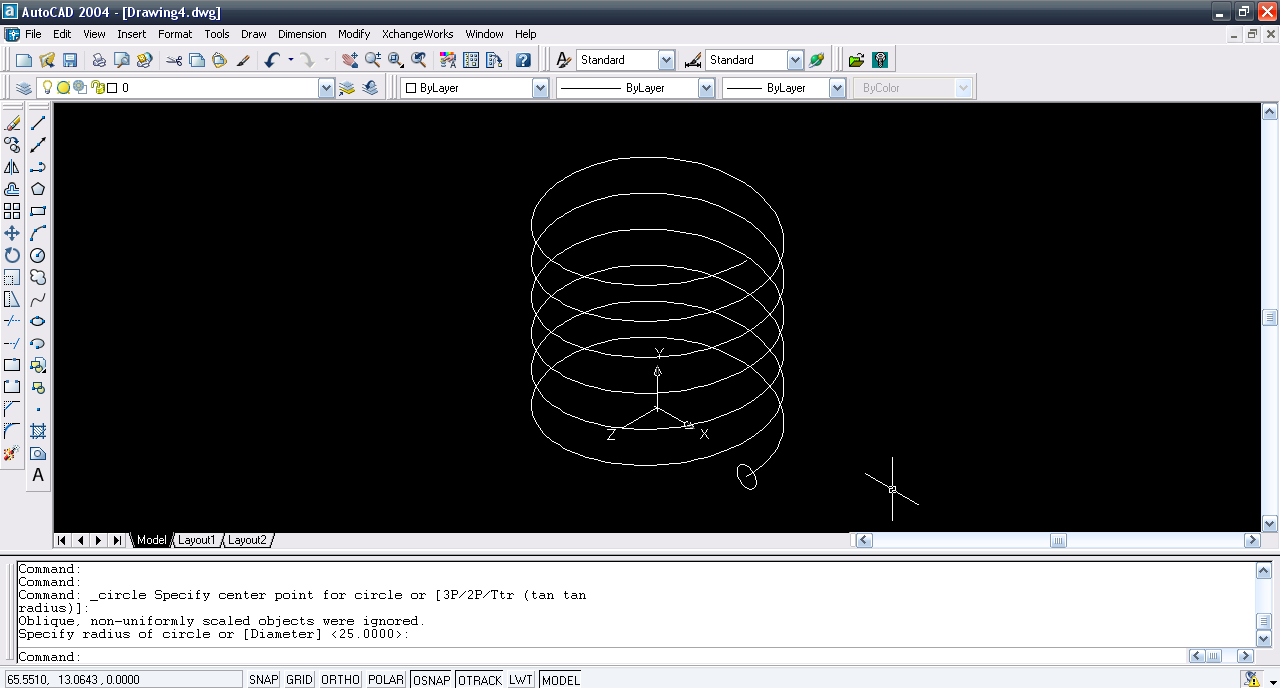20. ### Step 20:

Extrude command and then select the circle.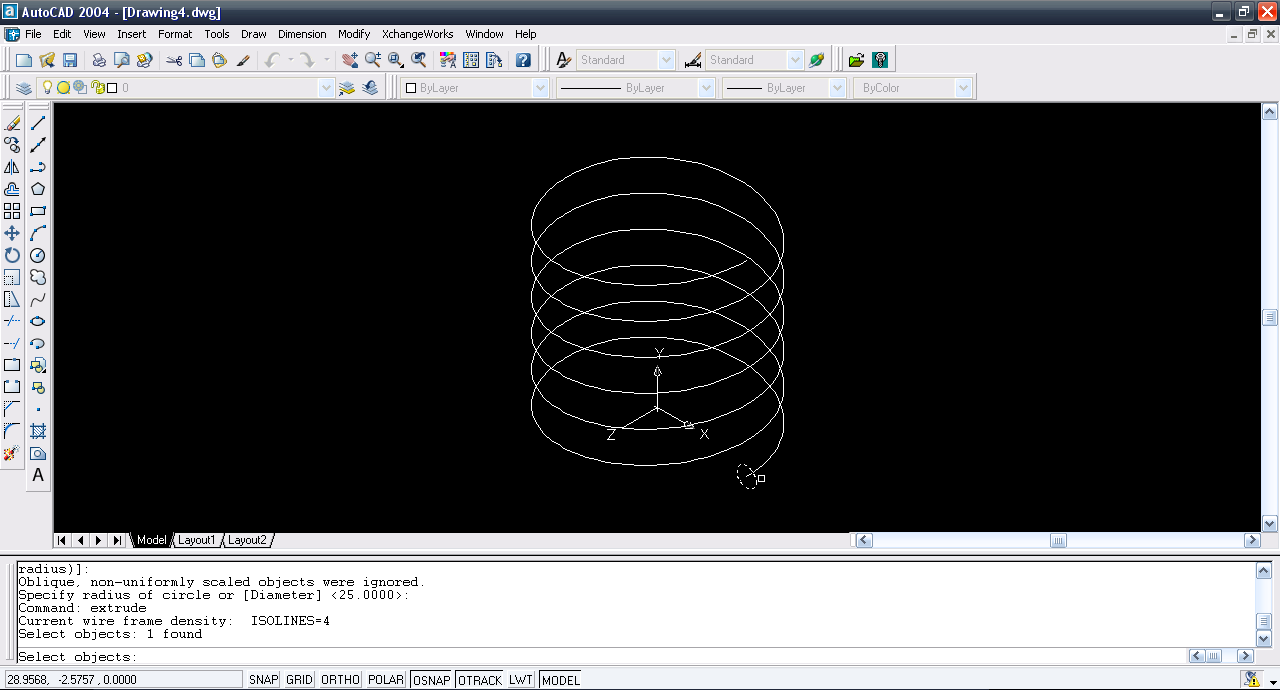21. ### Step 21:

Right click and then select path.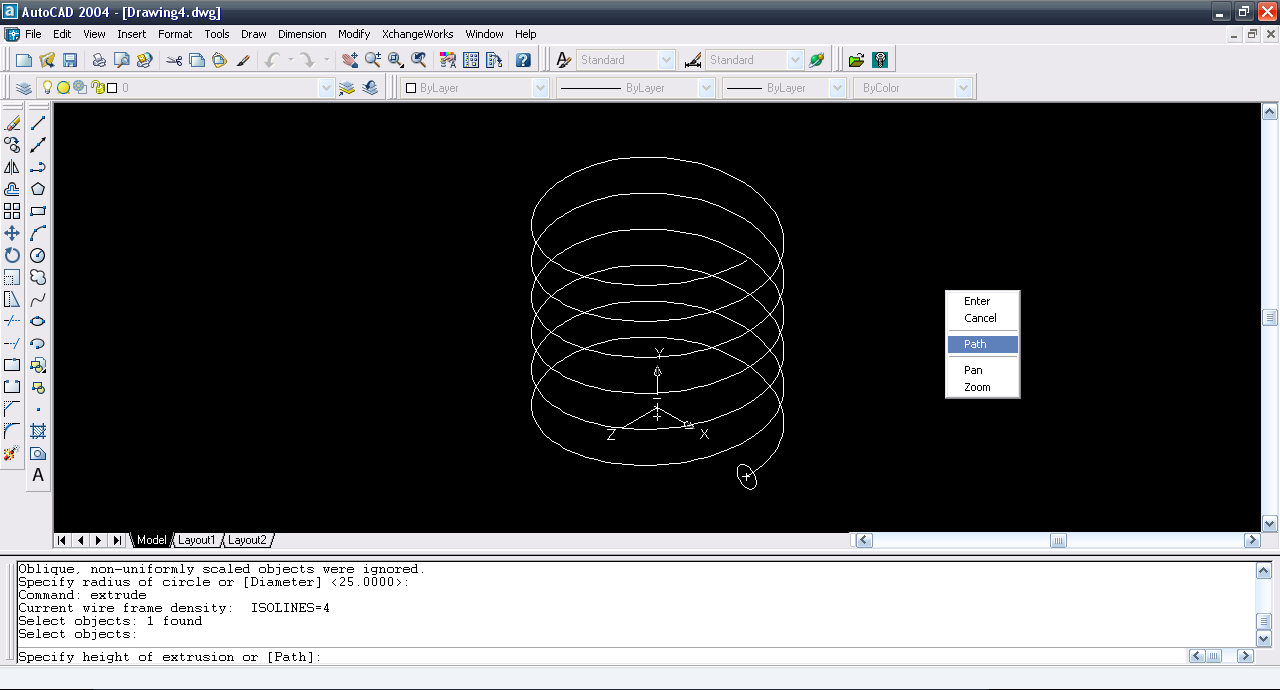22. ### Step 22:

Select the helix arc as the path and the part of the spring is generated.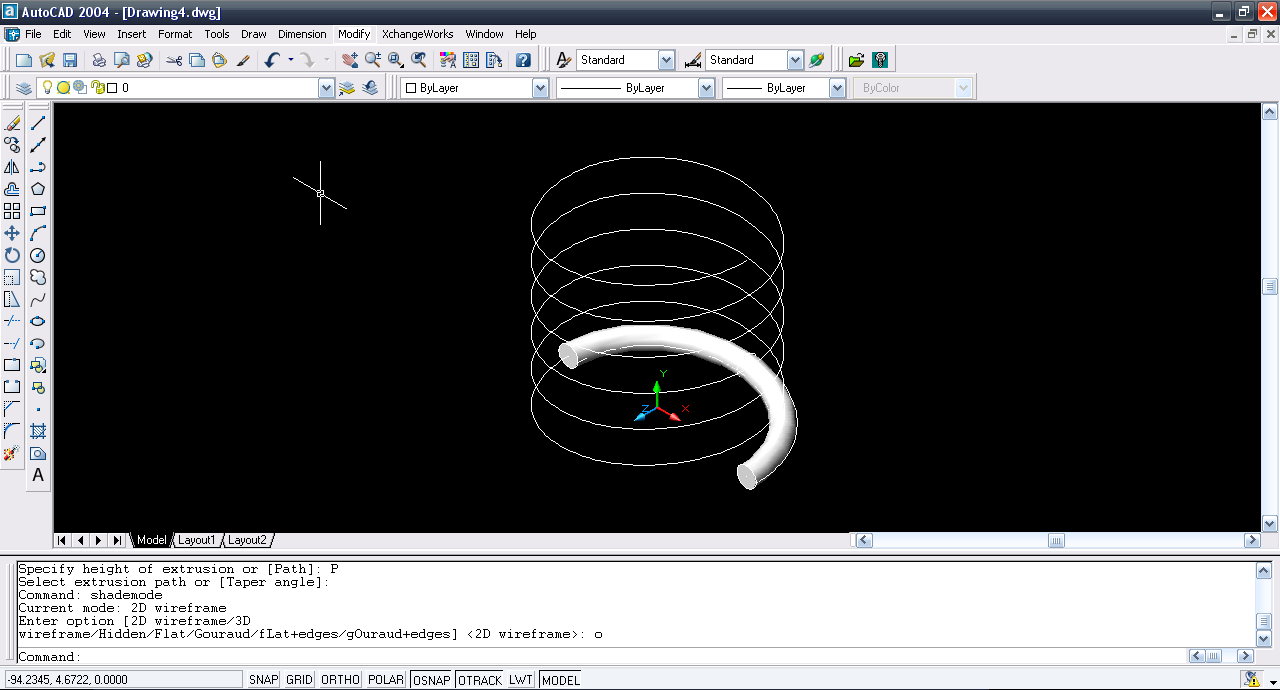23. ### Step 23:

Modify>>Solids Editing>>Extrude faces.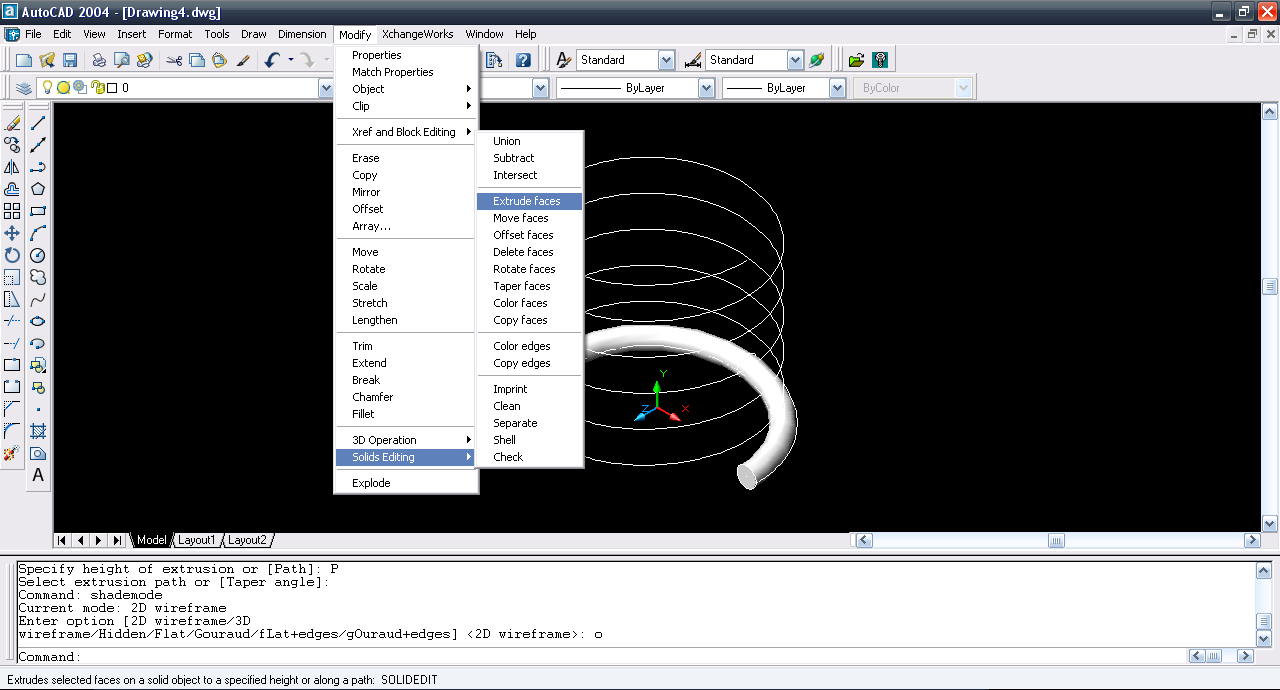24. ### Step 24:

Select the face of the helix.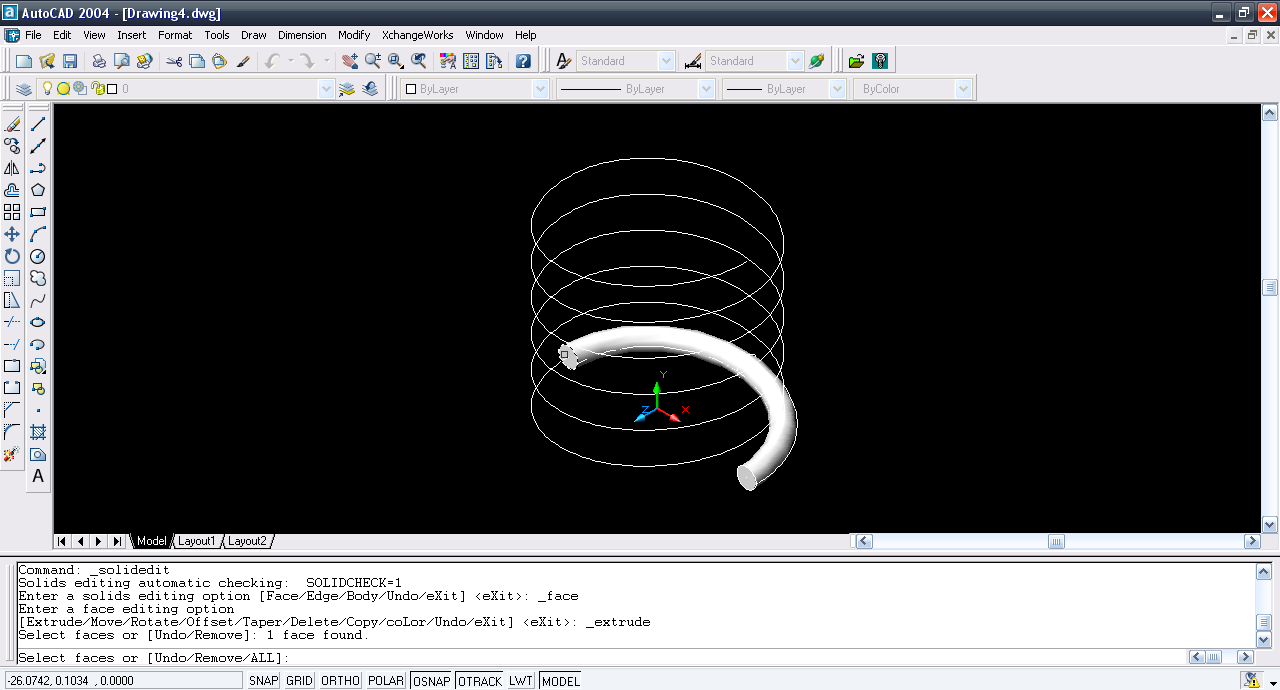25. ### Step 25:

Right click and then path.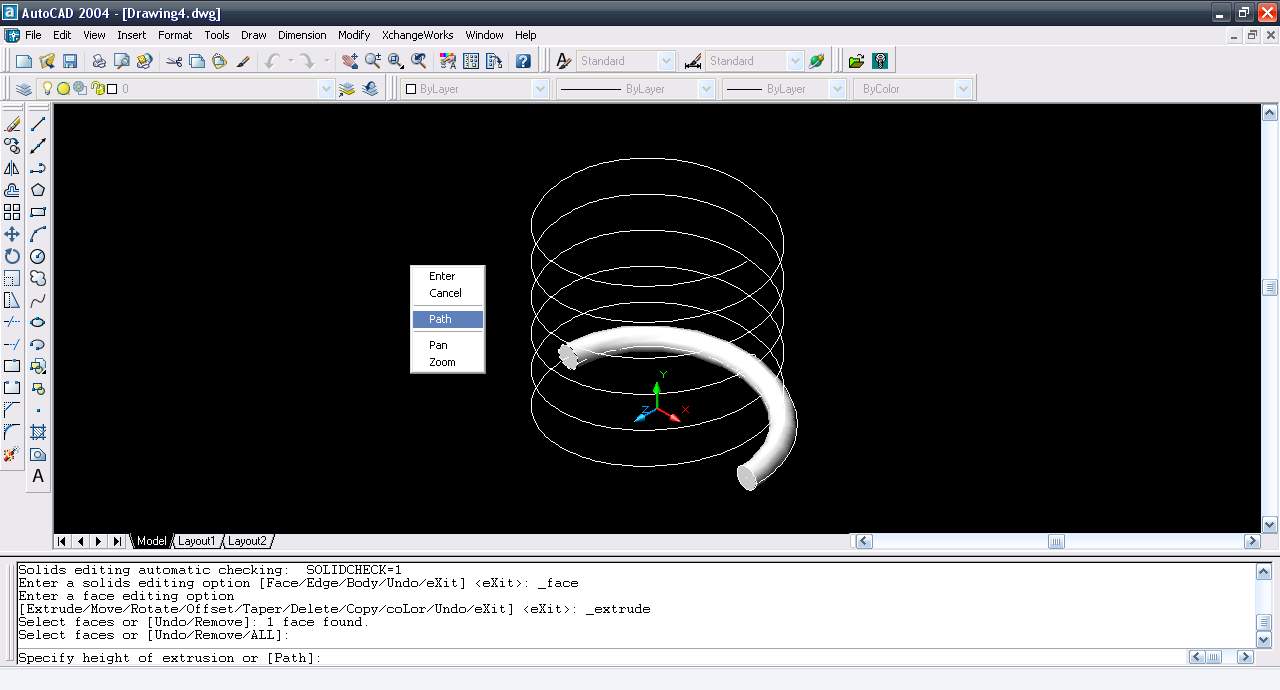26. ### Step 26:

Select the arc.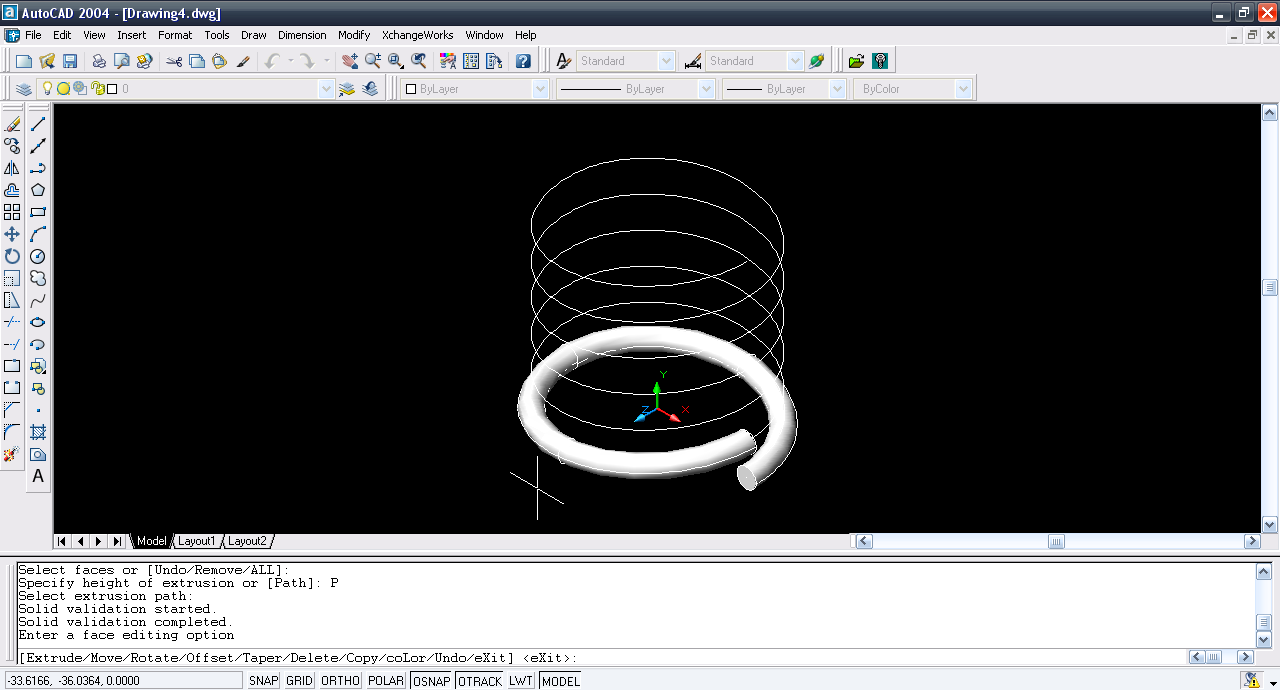27. ### Step 27:

Repeat the same step for the rest of the helix part and the spring is generated.